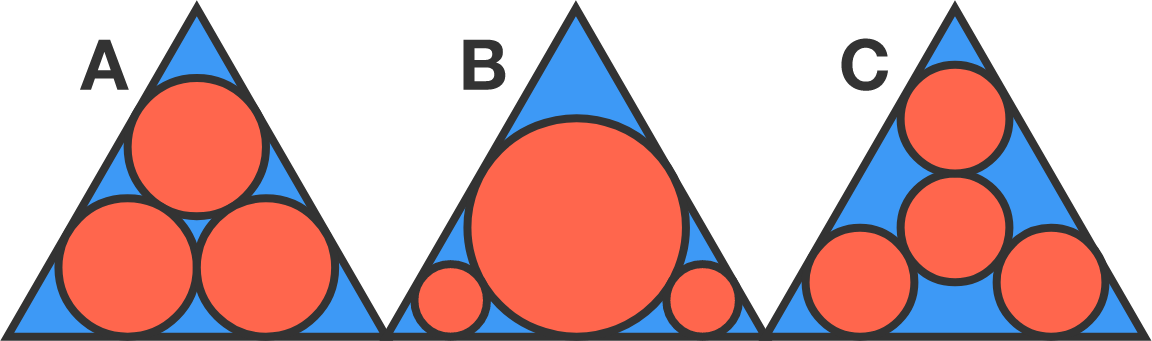# A Geometer's Fallacy

Geometry Level 1

Which of the following figures has the greatest area shaded in red?Assumptions:

• A, B, C are identical equilateral triangles. The circles of A are all congruent to each other, as are the circles of C.
• The red components are circles tangent to one another and to the triangle.

Note: If you get it wrong, don't worry! An Italian mathematician named Malfatti also got it wrong in the $19^\text{th}$ century.

×